Magneto-structural analysis of a coil by the sequential coupling method

HOME / Applications / Magneto-structural analysis of a coil by the sequential coupling method
WEBINAR
Simultaneous Wireless Information and Power Transfer
Thursday, January 27, 2022
Time
SESSION 1
SESSION 2
CET (GMT +1)
03:00 PM
08:00 PM
EST (GMT -4)
09:00 AM
02:00 PM
Used Tools:Electromagnetic force origin

The mechanical analysis of electrical conductors in terms of part deformation, acoustic and vibration characterization is a complex modeling problem. These phenomena arise mostly due to the ferromagnetic-part and coil conductor deflection. This example describes a way to analyze deformation of a current carrying conductors in a steady electromagnetic field. Lorentz force arises in such conductors and it is responsible for their deformation.

Sequentially coupled magneto-structural analysis

A sequential coupling is a method for the analysis of linear fields. Magnetic field is solved separately and the results are then used for the mechanical analysis. EMS first computes the resultant Lorentz force and then applies it in the structural analysis which calculates the deformation of the conductor (the coil).

The model consists of an air-cored cylindrical coil in its own magnetic field. This field is created by the coil current of 4500 A.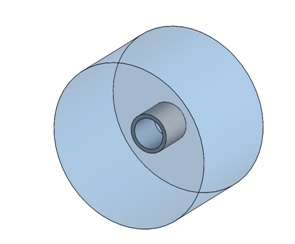Figure 1 - The solid coil surrounded by the air cylinder

Table 1 - properties of materials assigned to the model parts

 Components/Bodies Relative Permeability Electrical Conductivity (Mho/m) Elastic modulus (Pa) Poisson's ratio Mass   Density (Kg/m^3) Coil (copper) 1 5.840e+7 11e+10 0.3 8960 The air cylinder 1 0 Not required Not required Not Required

Fixed boundary condition is applied on the following two faces of the coil: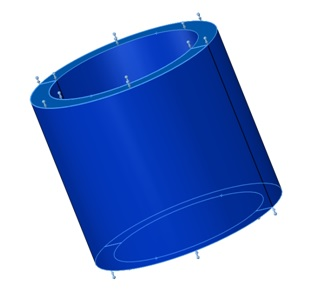Figure 3 -  Fixed boundary condition applied to the coil ends.

Meshing

EMS offers a very reliable and versatile meshing tools. Meshing a whole system requires using different mesh sizes depending on the required accuracy on each region of the model. In this example, it is important to apply fine mesh on the coil part to get accurate and detailed mechanical stress distribution.

Below, in Figure 4, is a section clipping plot of the mesh density in the coil and the air cylinder.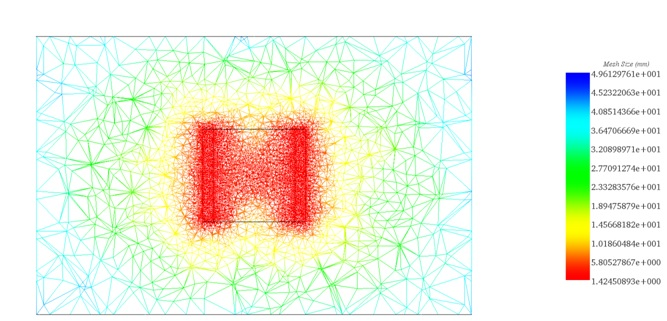Figure 4 - Section clipping plot of the mesh   density in the coil and the air cylinder

Magneto-structural results

Magnetic flux plot

Magnetic flux density is maximum inside the coil and it is parallel to the coil axis.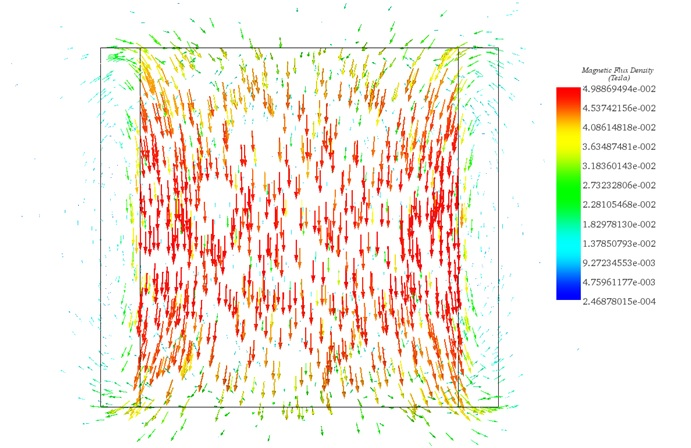Figure 5 - Field lines plot   of magnetic flux density in the coil and the air cylinder

Force density plot

The following figure shows distribution of the magnetic force density (Lorentz force) within the coil. Maximum force is shown to be in the tangential direction.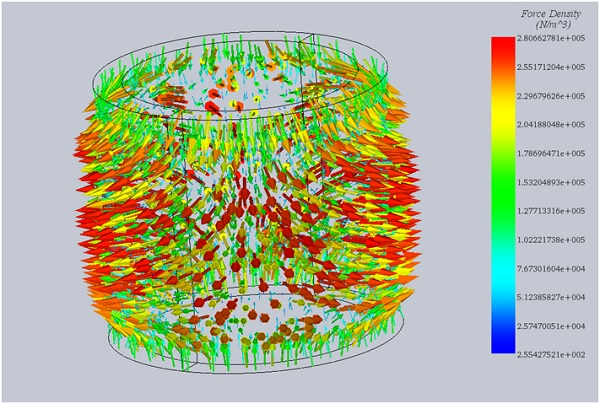Figure 6 - Lorentz force density distribution in the coil

Displacement plot

Lorentz force exerted on the coil leads to its deformation along the radial direction. Higher magnetic force may cause more deformation and thus should be controlled.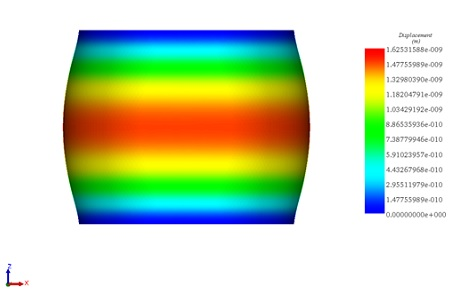Figure 7 - 3D plot of the resultant deflection in the coil

Stress Plot

Von Mises stress plot given below shows that maximum stress is on the ends of the coil. This stress is axial (along the coil axis). Another type of stress is the one exerted away from the ends of the coil. It is called hoop stress (tangential stress).

The user should be aware of superposition of those two types of stress while studying performance of coils under different operating conditions.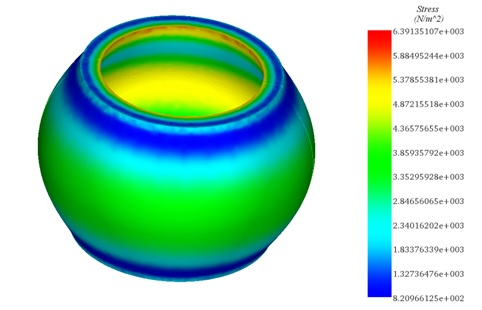Figure 8 - 3D plot of the resultant deflection in the coil

Table 2 - Comparison between EMS results and the reference

 EMS Results The Reference Results Maximum Magnetic Flux Magnitude (T) 4.98e-02 5.02e-02 Maximum Force Density (N/m3) 2.80e+05 2.49e+05 Maximum Resultant Displacement (m) 1.62e-09 1.66e-09

Note

Stress and displacement plots are scaled to show amplification of the deformation due to the magnetic field.

Conclusion

A sequential coupling between magnetostatic and stress analysis was established in a coil carrying a DC current. Obtained results show that Lorentz force acting on the coil gives rise to axial and hoop stresses. EMS magnetic and mechanical results are in very good agreement with those reported in the reference . In addition to structural coupling, thermal and motion coupling capabilities of EMS make it a multi physical environment that helps engineers to simulate several behaviours of their products.

References

:  A.P.S.BAGHEL,R.V. Thakar, S.V. Kulkarni, S.Chauhan. Linear Magneto-Structural Analysis by Sequential Coupling Method. 2009. National Conference on Technological Advances & Computational Trends in Electrical Engineering (TACT ).